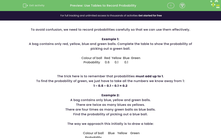# Use Tables to Record Probability

In this worksheet, students will practise recording probability using a table.Key stage:  KS 4

Year:  GCSE

GCSE Subjects:   Maths

GCSE Boards:   AQA, Eduqas, Pearson Edexcel, OCR,

Curriculum topic:   Probability

Curriculum subtopic:   Probability Basic Probability and Experiments

Difficulty level:#### Worksheet Overview

To avoid confusion, we need to record probabilities carefully so that we can use them effectively.

Example 1:

A bag contains only red, yellow, blue and green balls. Complete the table to show the probability of picking out a green ball.

 Colour of ball Red Yellow Blue Green Probability 0.6 0.1 0.1

The trick here is to remember that probabilities must add up to 1.

To find the probability of green, we just have to take all the numbers we know away from 1:

1 - 0.6 - 0.1 - 0.1 = 0.2

Example 2:

A bag contains only blue, yellow and green balls.

There are twice as many blues as yellows.

There are four times as many green balls as blue balls.

Find the probability of picking out a blue ball.

The way we approach this initially is to draw a table:

 Colour of ball Blue Yellow Green Probability

The issue we have is that we only know the number of balls relative to each other. To deal with this, we use algebra.

We can start by noticing that the smallest amount will be yellow, we call this x

If we have twice as many blue as yellow, we have 2x blue balls.

We have four times as many green as blue, this gives: 4 x 2x  =  8x green balls.

We can now fill in the table:

 Colour of balls Blue Yellow Green Probability 2x x 8x

Once again, we can look at the fact that the probabilities here add up to 1:

2x + x + 8x = 1

11x = 1

x = 0.091

Our last step is to find the probability we are asked for.

We are looking for the probability that we pick out a blue ball:

P(blue) = 2x

2 x 0.091 = 0.182

It seems a lot to remember, but it's easy if you take it one step at a time.

### What is EdPlace?

We're your National Curriculum aligned online education content provider helping each child succeed in English, maths and science from year 1 to GCSE. With an EdPlace account you’ll be able to track and measure progress, helping each child achieve their best. We build confidence and attainment by personalising each child’s learning at a level that suits them.

Get started